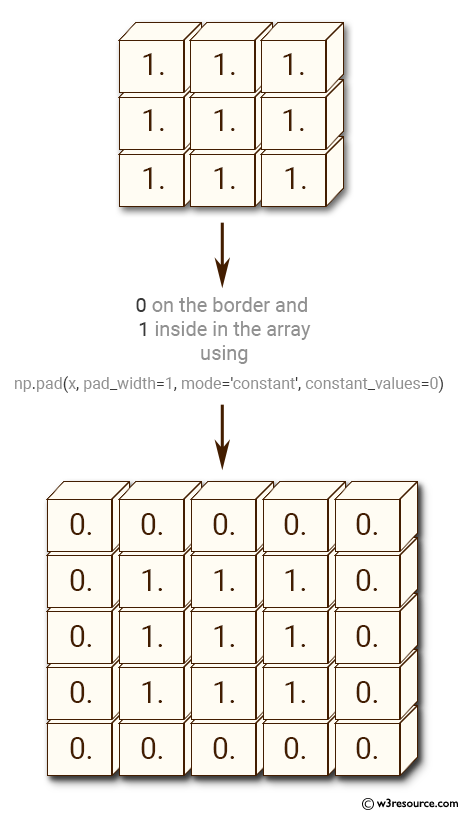﻿ NumPy: Add a border around an existing array - w3resource# NumPy: Add a border around an existing array

## NumPy: Array Object Exercise-9 with Solution

Write a NumPy program to add a border (filled with 0's) around an existing array.Sample Solution:-

Python Code:

``````import numpy as np
x = np.ones((3,3))
print("Original array:")
print(x)
print("0 on the border and 1 inside in the array")
print(x)
```
```

Sample Output:

```Original array:
[[ 1.  1.  1.]
[ 1.  1.  1.]
[ 1.  1.  1.]]
1 on the border and 0 inside in the array
[[ 0.  0.  0.  0.  0.]
[ 0.  1.  1.  1.  0.]
[ 0.  1.  1.  1.  0.]
[ 0.  1.  1.  1.  0.]
[ 0.  0.  0.  0.  0.]]
```

Python Code Editor:

Have another way to solve this solution? Contribute your code (and comments) through Disqus.

What is the difficulty level of this exercise?

Test your Python skills with w3resource's quiz

﻿

## Python: Tips of the Day

List comprehension:

```>>> m = [x ** 2 for x in range(5)]
>>> m
[0, 1, 4, 9, 16]
```